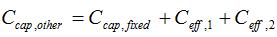# HOMER Pro 3.9

 Navigation: Glossary Other Capital CostType: Output Variable Units: \$ Symbol: Ccap,other

HOMER uses the 'other capital cost' to account for the system fixed capital cost and, if any primary load efficiency measures are in effect for the current system configuration, the capital cost associated with those efficiency measures.

The 'other capital cost' appears in the Cash Flow Details Table.

HOMER uses the following equation to calculate the other capital cost:where: Ccap,fixed Ceff,1 = cost of efficiency measures (if any) for primary load 1 [\$] Ceff,2 = cost of efficiency measures (if any) for primary load 2 [\$]

See also

System Fixed Capital Cost

Other Operation and Maintenance Cost